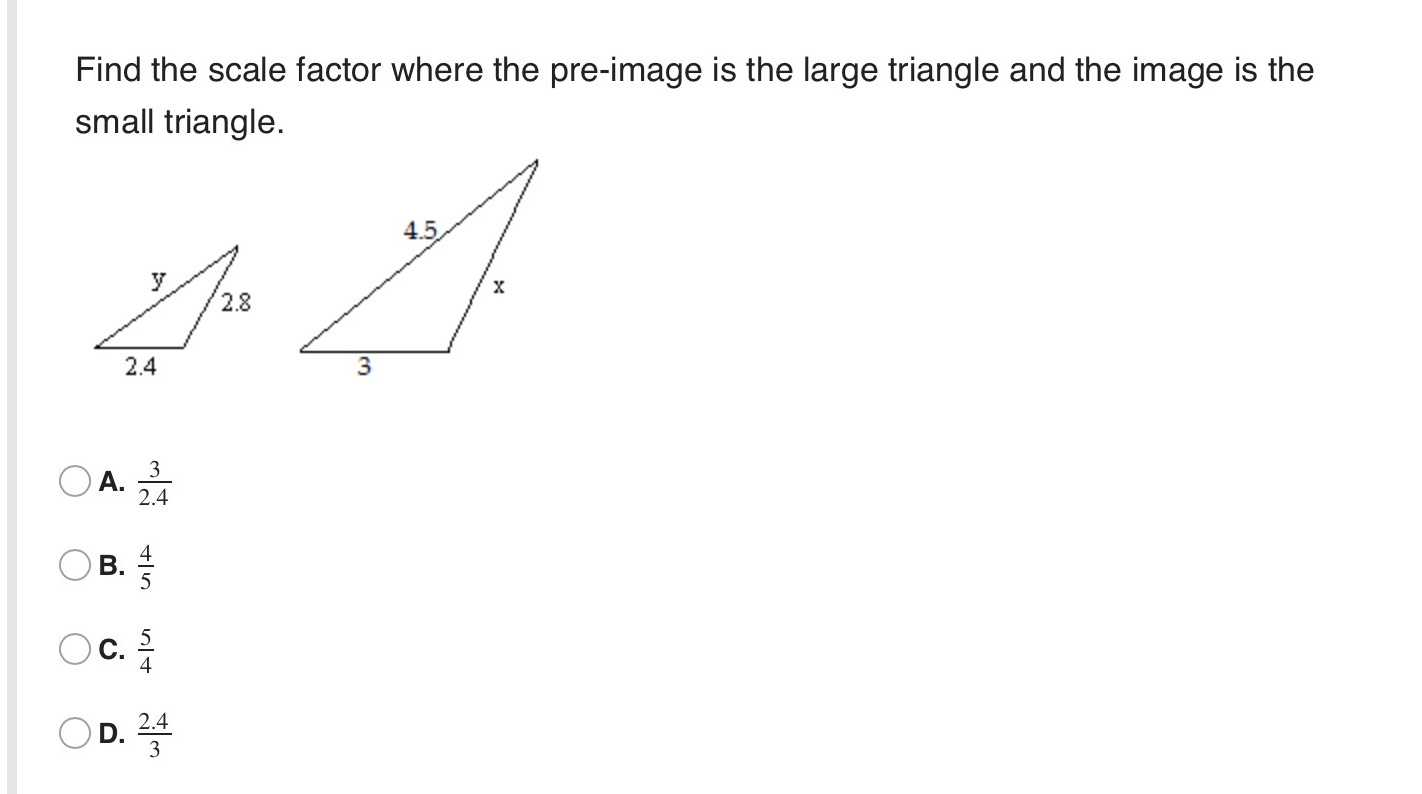### Still have math questions?

Trigonometry
QuestionFind the scale factor where the pre-image is the large triangle and the image is the small triangle.

A. $$\frac { 3 } { 2.4 }$$

B. $$\frac { 4 } { 5 }$$

C. $$\frac { 5 } { 4 }$$

D. $$\frac { 2.4 } { 3 }$$